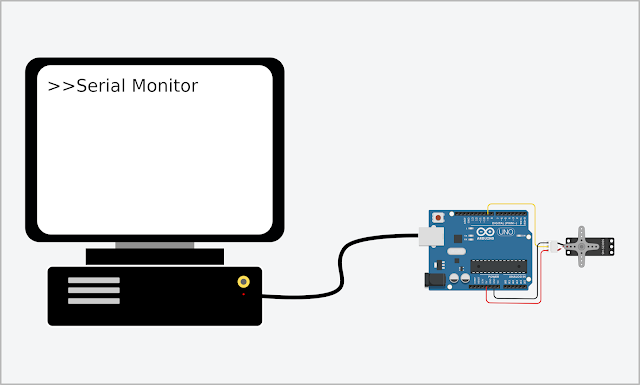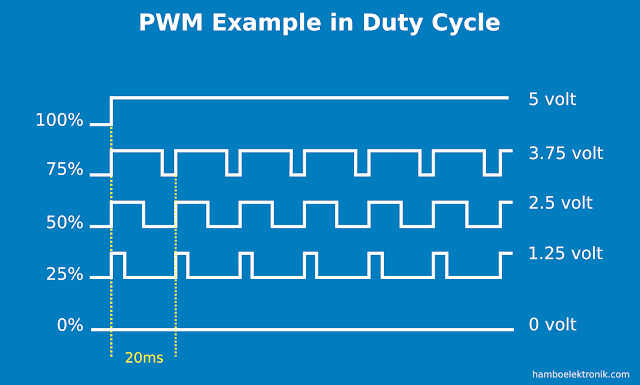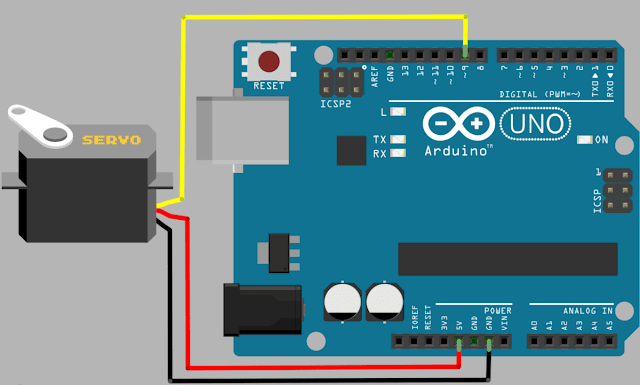How to Control Arduino Servo Motor Through Serial Monitor

What is Servo Motor?

How to Control an Arduino Servo Motor Via Serial Monitor - In some project applications, we will control or control a servo motor via serial communication. Using Arduino we can easily do this.Motor Servo Arduino Serial

Servo is a motor that can be controlled to rotate to a certain level. In general, this servo motor can rotate from 0 degrees to 180 degrees.

Some can rotate up to 360 degrees. This motor has three wires. Two of them are + and - and one is for data. The data cable is used to control the rotation of the servo motor.

This servo motor is a major component in robots, servo robots, RC cars and RC planes. To control this servo motor is very easy.

With the help of the Servo.h library that has been provided on the Arduino IDE, we simply enter the number of degrees of rotation of the motor, the arduino servo motor will rotate according to the number of degrees we want.

Popular servos used for this tutorial are Servo Tower Pro MG996R, Tower Pro SG5010, AX12 Dynamixel, Tower Pro SG90, Tower Pro MG90, Savox 1268SG, Savox 1267, Savox 1251, Savox 1256, Savox 1258.

PWM Concept in Servo Motor

Servo motors work based on pulse width modulation (PWM). PWM is a method of getting an analog value or voltage from a digital value. Voltage 0-5 volts is described as a digital value 0-255.

However, to describe how big the cycle is, the PWM servo uses percent. Consider the following example:

Arduino pins only produce a maximum voltage of 5 volts. So, a small pulse width will produce a voltage swing of 0-5 volts.The wider the pulses in the signal cycle, the larger the resulting analog voltage. Writing the value if we use the "analogWrite()" command is as follows:

analogWrite (0) = 0% = 0 volts
analogWrite(64) = 25% = 1.25 volts
analogWrite (127) = 50% = 2.5 volts
analogWrite (191) = 75% = 3.75 volts
analogWrite (255) = 100% = 5 volts

The frequency used in the cycle is 50Hz.

How to Rotate the Servo to a Specific Position

Now we will try to rotate the Arduino servo motor. Generally to drive a servo motor, a maximum cycle time of 2ms is required. 1ms for 0°, 1.5ms for 90° and 2ms for 180°. Look at the following image:We will discuss the program that will be used in this tutorial, but first please connect Arduino and Servo Motor as shown in this image.D. Program

1. Basic Code

The command to drive this servo motor on the Arduino IDE is "analogWrite()". Here is a basic servo program:

 1 2 3 4 5 6 7 8 9 10 11 #include Servo myservo; void setup() { myservo.attach(9); //Pin PWM myservo.write(90); //rotate 90 degrees } void loop() {}

2. Converting Char to Int

If we send a serial monitor value, 90 for example, with the aim of rotating the servo to 90 degrees, this will not work. Because the value we receive from the serial monitor is a character or data type char.

While the value we need to determine the position of the servo motor is a value with an integer data type.

To change the data type from char to int we can use the command "Serial.parseInt()". For a tutorial on converting data types from a serial monitor, read here.

Following are the basic commands to convert values ​​from serial monitor to integer values:

 1 2 3 4 5 6 7 8 9 10 11 void setup(){ Serial.begin(9600); } void loop(){ while (Serial.available() != 0){ int val = Serial.parseInt(); Serial.println(val); delay(20); } }

3. Servo Control Using Serial Monitor

We will use arduino as the basic servo controller program and convert the above values ​​to drive the servo motor, as follows:

 1 2 3 4 5 6 7 8 9 10 11 12 13 14 15 16 17 #include Servo myServo; void setup() { Serial.begin(9600); myServo.attach(9); } void loop() { while (Serial.available() != 0) { int val = Serial.parseInt(); Serial.println(val); myServo.write(val); delay(5); } }

The above program has been tried and worked. The above is a basic guide on how servos work.

If you understand this, chances are you can understand how to control high-speed, high-speed servo and hybrid servo drives such as 5kg servo motor, 15kg servo motor, 20kg servo motor, 30kg servo motor, 100kg servo motor.

Famous manufacturers such as Tecnica servo, Bosch servo, Lenze servo, Elmo Motion, Beckhoff Servo Drive, Omron Servo, Parker Servo, Lenze 9400, Kuka servo motor, Mitsubishi servo drive, Siemenst sinamic v90, ABB servo motor, Allen Bradley servo motor, Hiwin servos, Panasonic mbdjt2210 servo drives.

Thank you for visiting Chip Piko's website. May be useful.